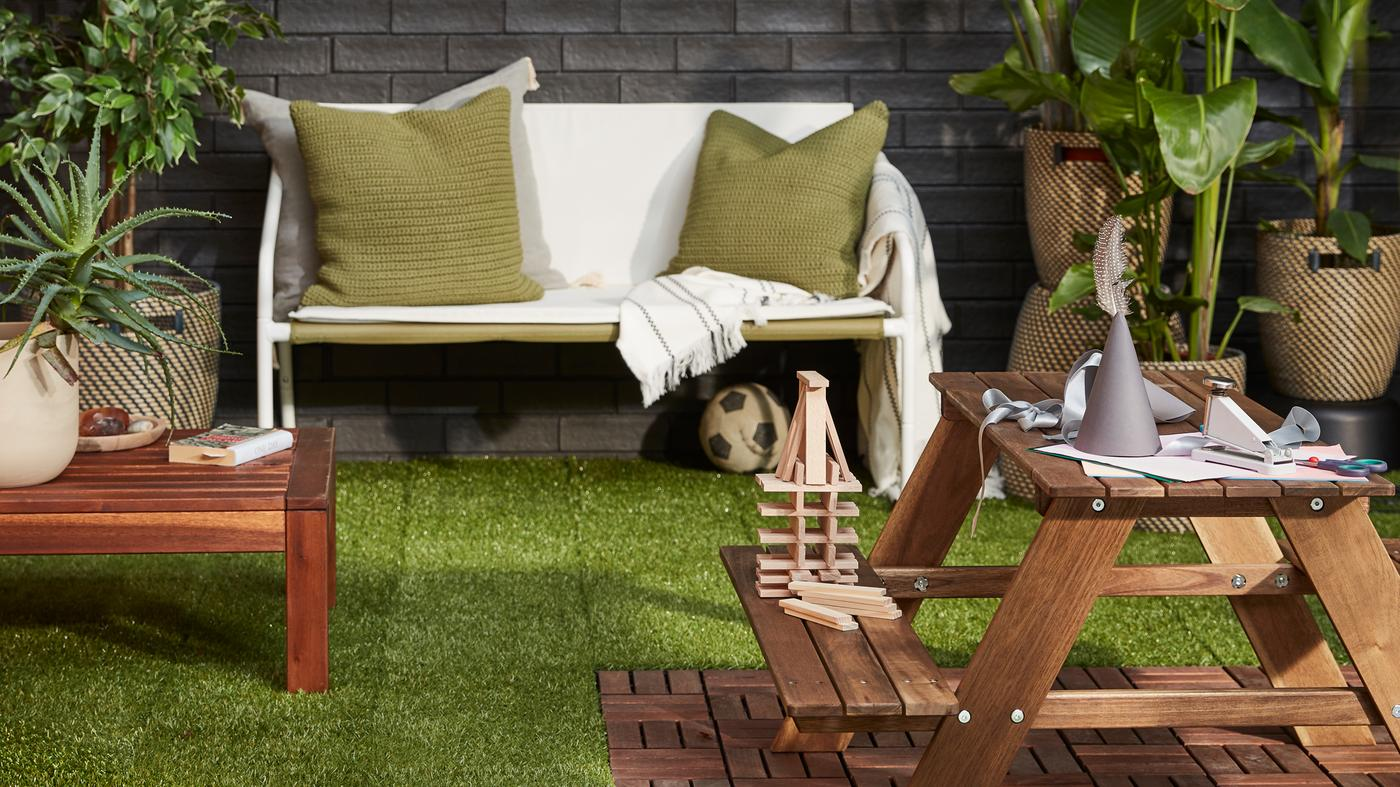# How to calculate how much decking you need

Creating an inviting outdoor space with floor decking that clicks together is easy when you’ve worked out how much flooring to buy. Check out our simple guide below to find out how much flooring you need.## 1. Find the surface area

Start by measuring the length and width of your outdoor space.

## 2. Calculate how many packages you need

Please enter the dimensions measured above into the tile calculator below. If you already know the total space area, enter it directly in the "area" column of the calculator by numbers.

### {{title}}

{{metric_dims}}
{{metric_dims}}
{{or}}
{{metric_area}}

The calculation result is a half-down value and is calculated below the required quantity. Try to buy additional packages by measuring the remaining area.